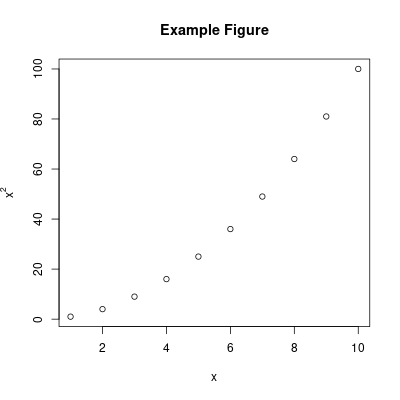I use R a lot. It's one of the primary tools I use in my day job as a scientist analyzing large datasets. If you use LaTeX with R (as I often do), you probably use Sweave to interleave R output and figures with your text describing those figures using the noweb method of literate programming.

Sweavealike is a plugin for IkiWiki that tries to do some of the useful things for IkiWiki that sweave does for R and LaTeX.

You use it like the following:

``````[[!sweavealike  echo=1 code="""
a <- 1
a <- a + 10
print(a)
"""]]
``````

which produces this result when run:

``````> a <- 1
> a <- a + 10
> print(a)
 11
``````

You can also generate figures with it:

``````[[!sweavealike  fig=1 echo=1 results="hide" code="""
plot(1:10,(1:10)^2,xlab="x",ylab=expression(x^2),main="Example Figure")
"""]]

> plot(1:10,(1:10)^2,xlab="x",ylab=expression(x^2),main="Example Figure")
``````The plugin itself uses the neat Statistics::R perl module to handle all of the heavy lifting. I personally plan on using this plugin to help write some more entries in my learning R series of posts that I'm beginning to work on. Hopefully I'll find and fix most of the bugs as I embark on that process so anyone else who uses the plugin won't, but feel free to e-mail me if something isn't working as it should.

Finally, you shouldn't run this plugin on a publicly editable IkiWiki instance, because that would be a trivial local user exploit as R can run arbitrary code, read and write to arbitrary files, exhaust all memory, etc.